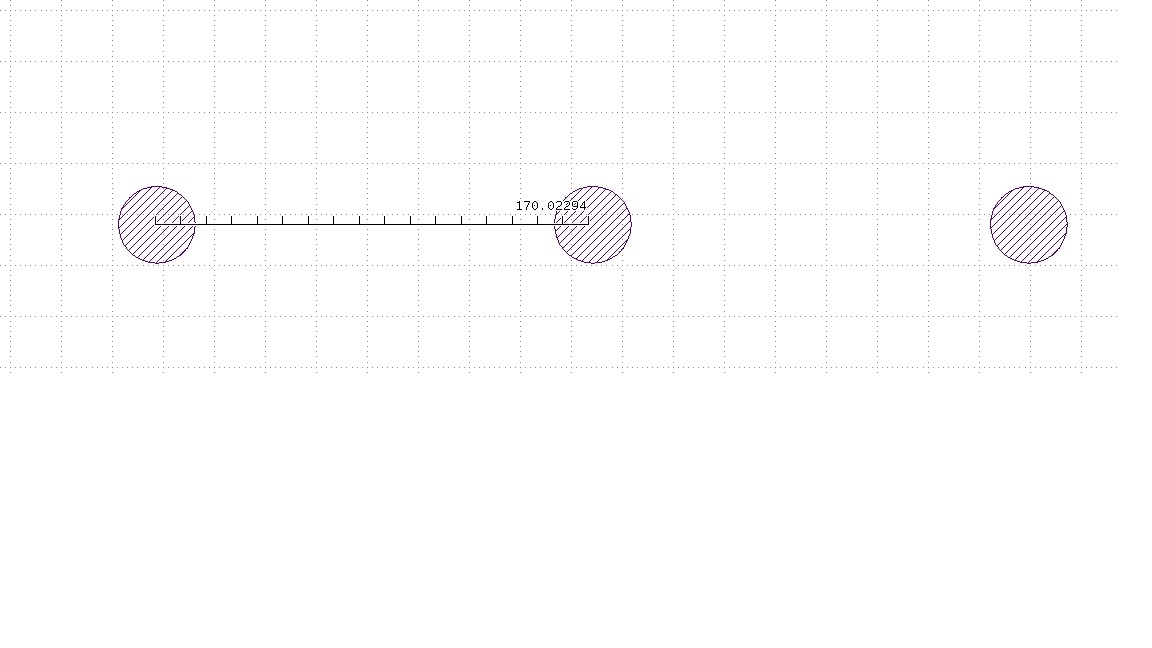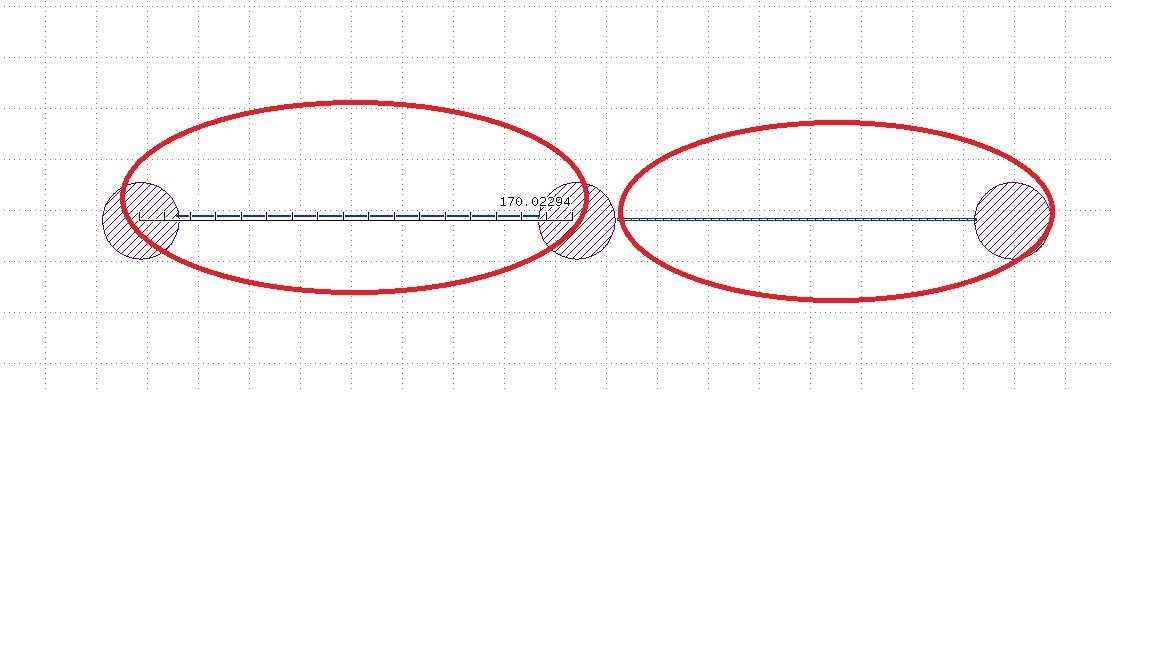# How to get the min. pitch value in layout?

Hi sir,
I want to check how the min. pitch in the layout .
and here is my Ruby code, but when I get the array length , I get a wrong value.
(totally , "UBMspace" layer may have 3000 patterns , but the "outputdataX" array only have around 497 value.
so when the case have a few of patterns in the check layer (as the sample , 152/1 layer) , I can base on this code to get the answer.
But , when the number of pattern more than (~500) , the code will provide a wrong answer.
Thanks.

``````    UBM=input(152,1)
UBM_PitchValue=200
UBM.middle.size(0.5).output(300,0)
UBM_Layer=input(300,0)
UBM_Layer.space(UBM_PitchValue.um).output(300,1)
UBMspace=input(300,1)
outputdataX = Array.new
UBMspace.each do |obj|
outputdataX << Math.sqrt(((obj.bbox.height.round(3)* obj.bbox.height.round(3))+ (obj.bbox.width.round(3)* obj.bbox.width.round(3))))
end
minValue=outputdataX.min

UBM_Layer.space(minValue.um).output(300,2)
puts minValueCheck
puts outputdataX.length()
``````

• Sorry, I don't get it.

You're computing the diagonal of the bounding boxes (BTW: why round(3)?) and put them into an array. How is the array length related to min pitch? And what is minValueCheck?

Matthias

• Hi Matthias,
Thanks for your reply , I need to check what the min. pattern pitch as picture below.so , we have 2 ways to do that.
1.get the patternBBox in X and Y by each pattern, and check the space between (X1,Y1) , (X2,Y2)
but it will take too long process time.

so , I using another ways -- using a defalut value , in this scribe case it is UBM_PitchValue=200.
and using space functon to check the pattern space between center to center.

if the min. space >= 200, (I get not space check result , I wil make another the check value such as 300)
the space function can check the pattern out of rule (space <200m)

I can output the check box by bboxso , I want to base on the new pattern check check what the size is and collect the value in array "outputdataX "

finally , I get the min. value in this array , should I get the answer.

cause the coordinate grid issue , such as (0.0005,0.0009) , I want to skip it , so I using round(3) to skip the small value...

in this scribe , when the counts of pattern <50 , look like I can get answer , But when the amount of pattern >5000 , I can't get the correct answer , it is why I post the question .
Thanks very much for your help.

• If the pads all have a common master then could
you not just collect the origin X,Y and do (X2-X1),
(Y2-Y1) origin-origin saving the least delta found,
dumb-loop-sort-style? Pitch is center to center.

Of course this might fall apart if you have a mix
of pad geometries, or errors that violate min pitch
(maybe a smarter implementation would also check
and tabulate anything below some input-ed pad
spacing groundrule, "set the floor" like?).

• @jiunnweiyeh

The usual way I do that is to sort all center values (bbox.center()) by y coordinate, pick all with the same y coordinate, sort by x and take x[n+1] - x[n] as the pitch. Then look for the smallest pitch.

Matthias